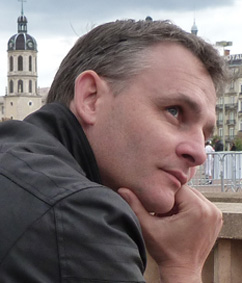• A
• A
• A
• АБВ
• АБВ
• АБВ
• А
• А
• А
• А
• А
Обычная версия сайта

Frederic Mangolte

Frederic Mangolte (l'Université d'Angers) посетил Лабораторию алгебраической геометрии и ее приложений  в ноябре 2016.

2 ноября он выступил с докладом Fake real planes: exotic affine algebraic models of R^2 на еженедельным семинаре Лаборатории

Abstract: We study topologically minimal complexifications of the Euclidean affine plane R² up to isomorphism and up to birational diffeomorphism. A fake real plane is a smooth geometrically integral surface S defined over R such that: The real locus S(R) is diffeomorphic to R^2; The complex surface S_C(C) has the rational homology type of A^2_C.; S is not isomorphic to A^2_R as surfaces defined over R.  The analogous study in the compact case, that is th classification of complexifications of the real projective plane P^2(R) with the rational homology of the complex projective plane is well known: P^2_C is the only one. We prove that fake real planes exist by giving many examples and we tackle the question: does there exist fake planes S such that S(R) is not birationally diffeomorphic to A^2_R(R)? (Joint work with Adrien Dubouloz.)

Анонс доклада (DOC, 51 Кб)Нашли опечатку?
Выделите её, нажмите Ctrl+Enter и отправьте нам уведомление. Спасибо за участие!
Сервис предназначен только для отправки сообщений об орфографических и пунктуационных ошибках.